# RS Aggarwal Solutions for Class 10 Maths Chapter 2 Polynomials Exercise 2A

RS Aggarwal Solutions for Class 10 Maths Chapter 2 Polynomials, contains solutions for all exercise 2A questions. RS Aggarwal solutions on exercise 2a is based on polynomials, its classifications and how to find zeros of a polynomial. Students can download the RS Aggarwal Solutions of Class 10 mathematics to furthermore understand the concept.

### Download PDF of RS Aggarwal Solutions for Class 10 Maths Chapter 2 Polynomials Exercise 2A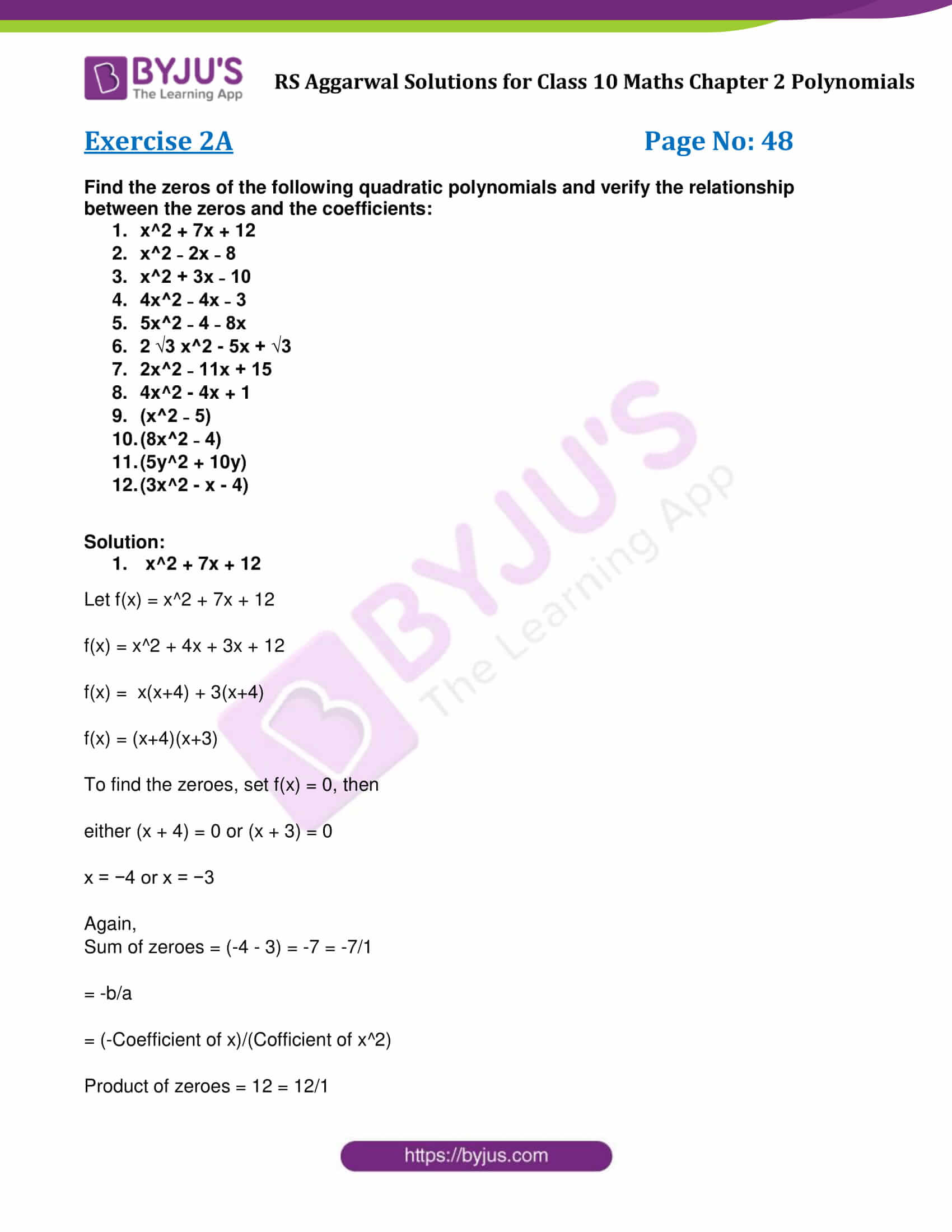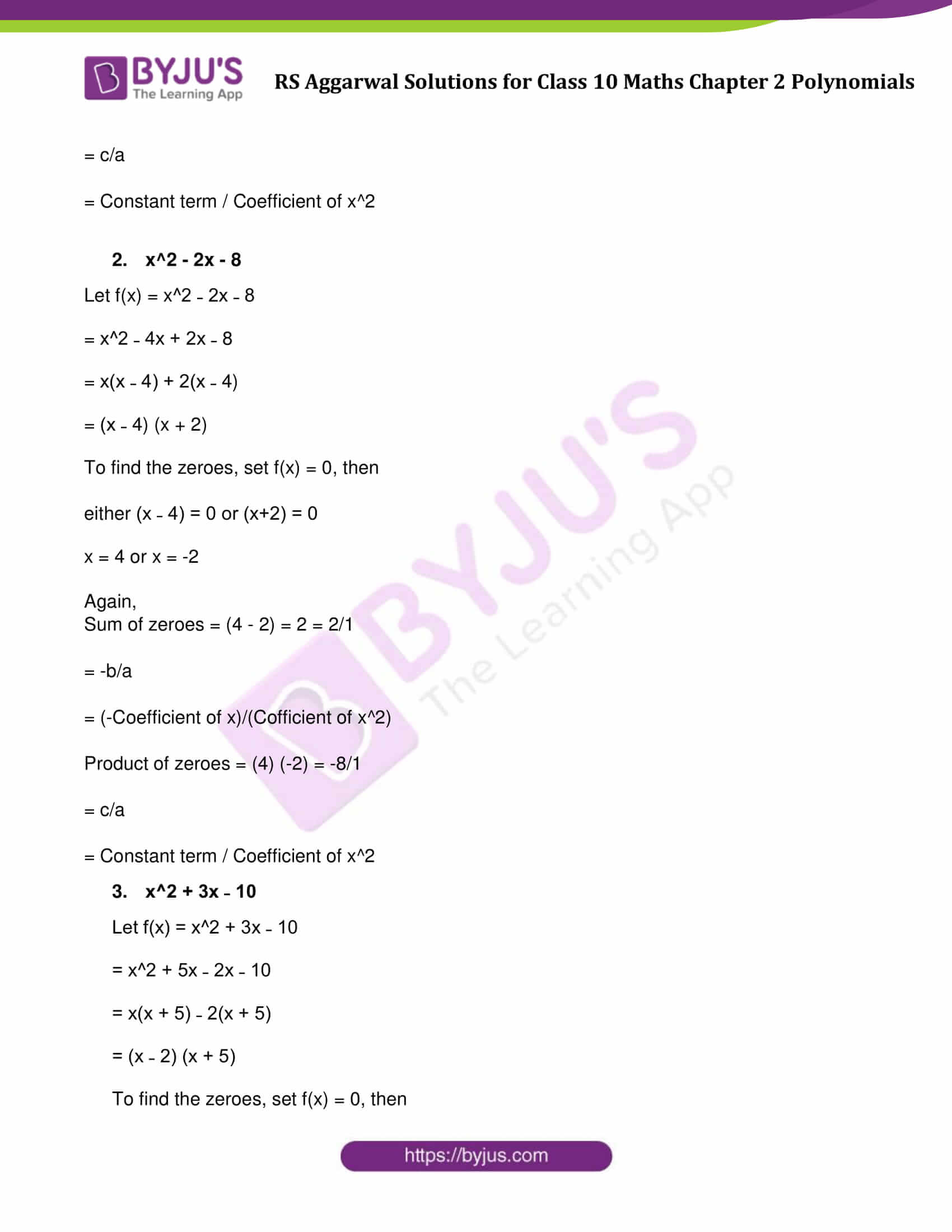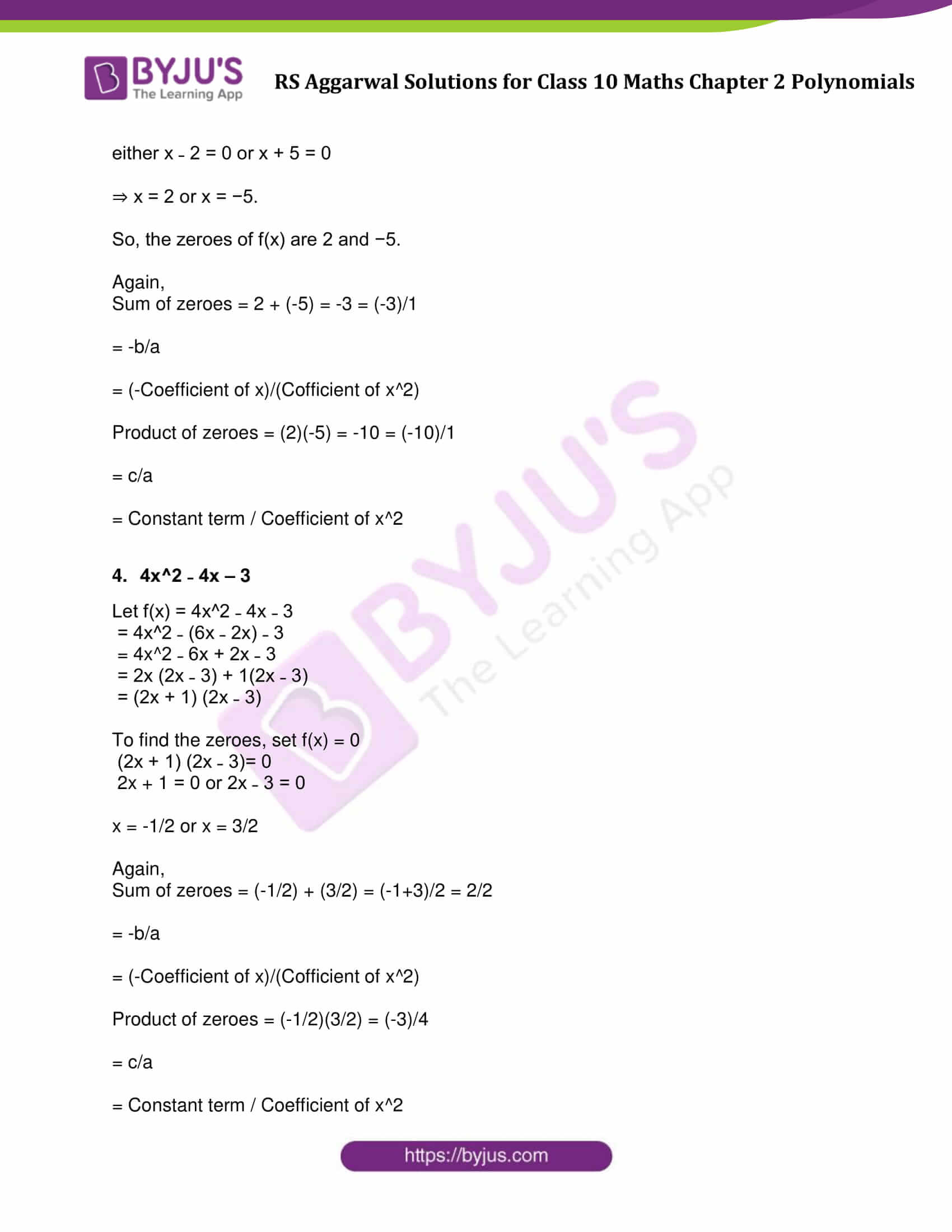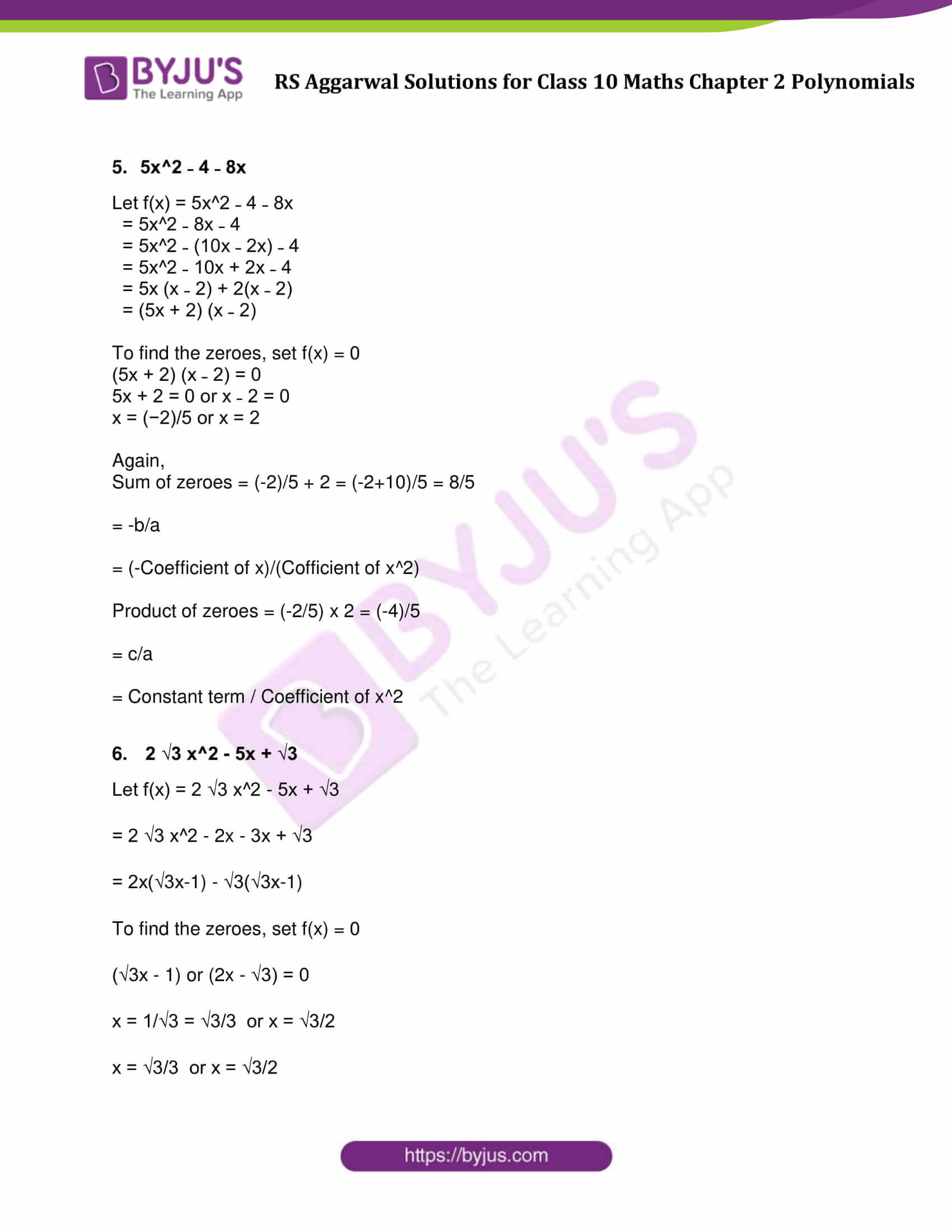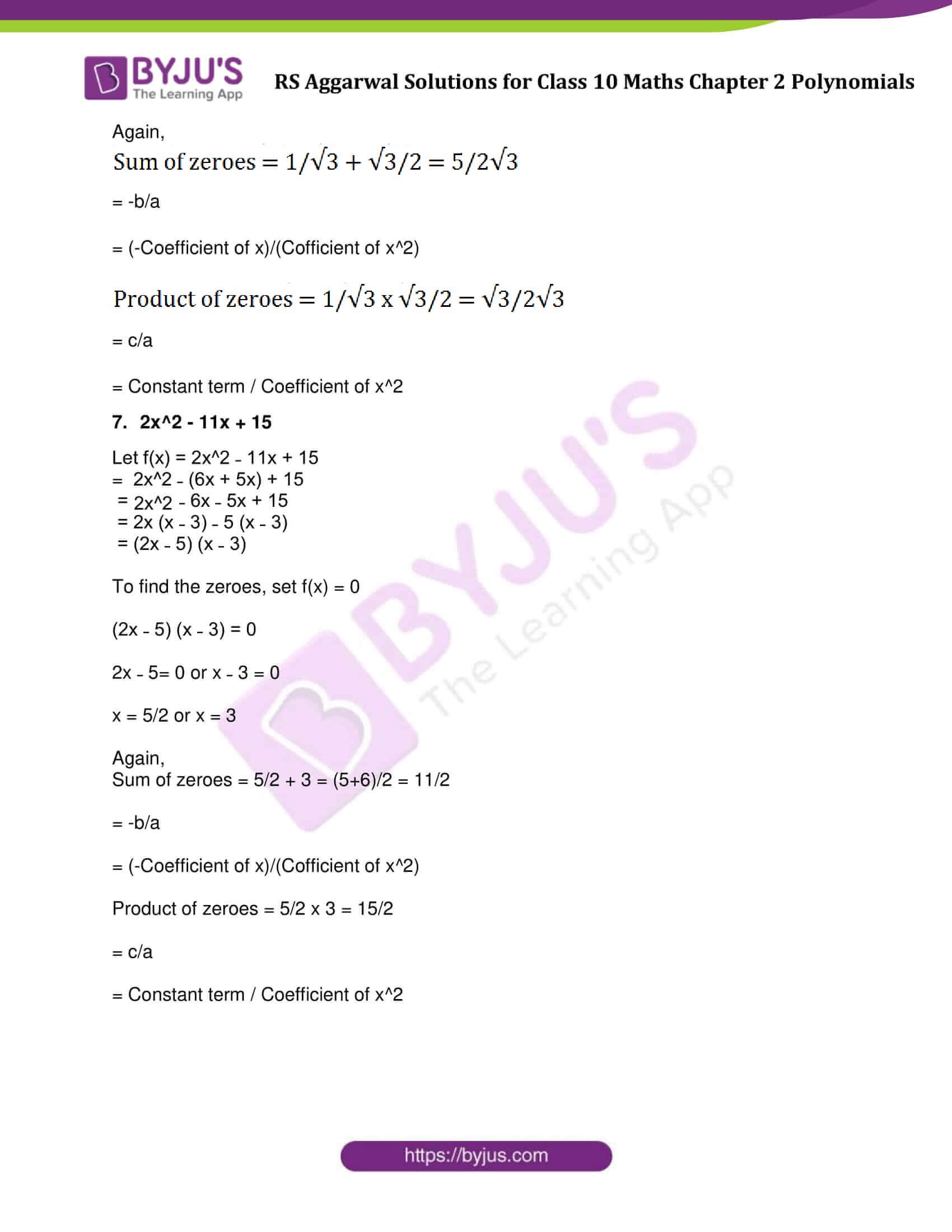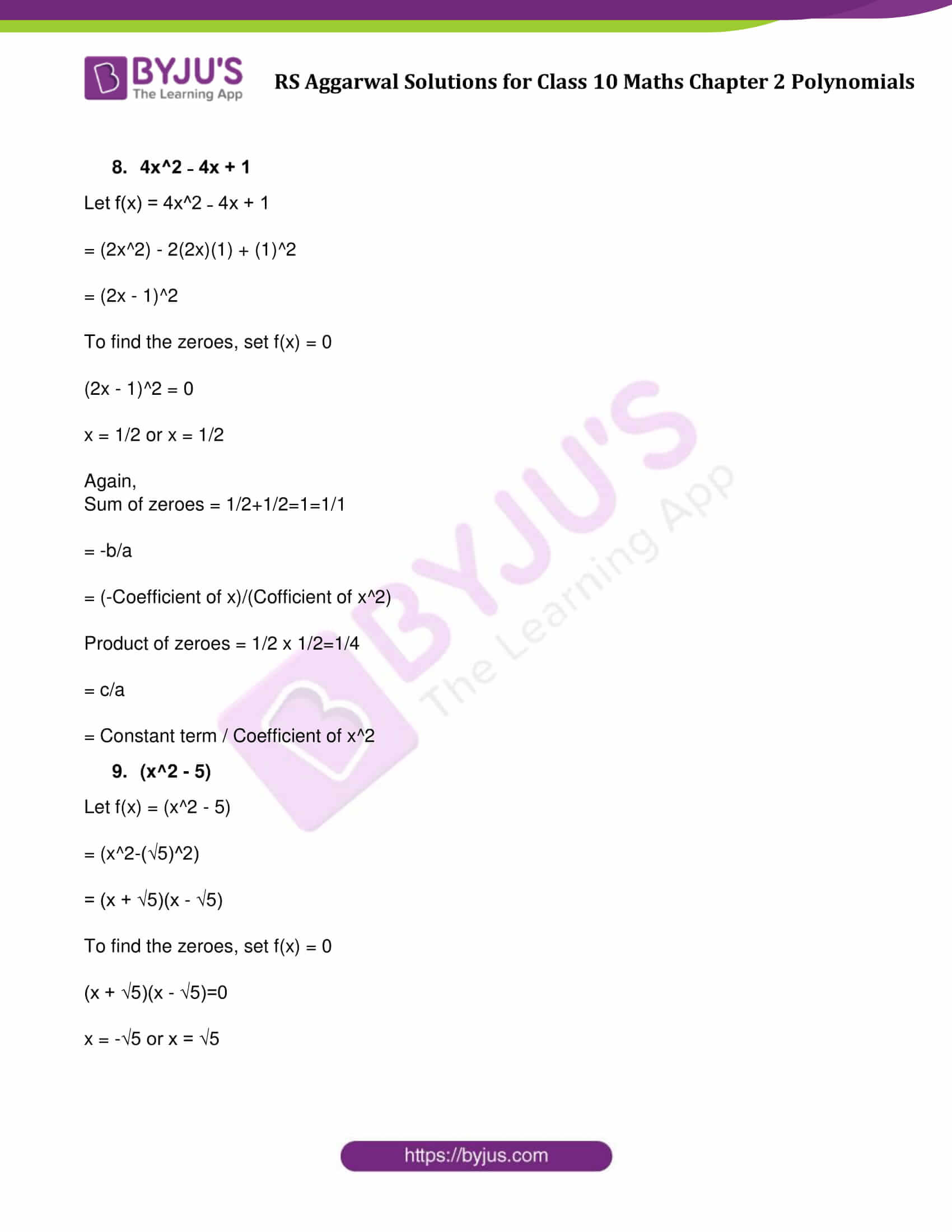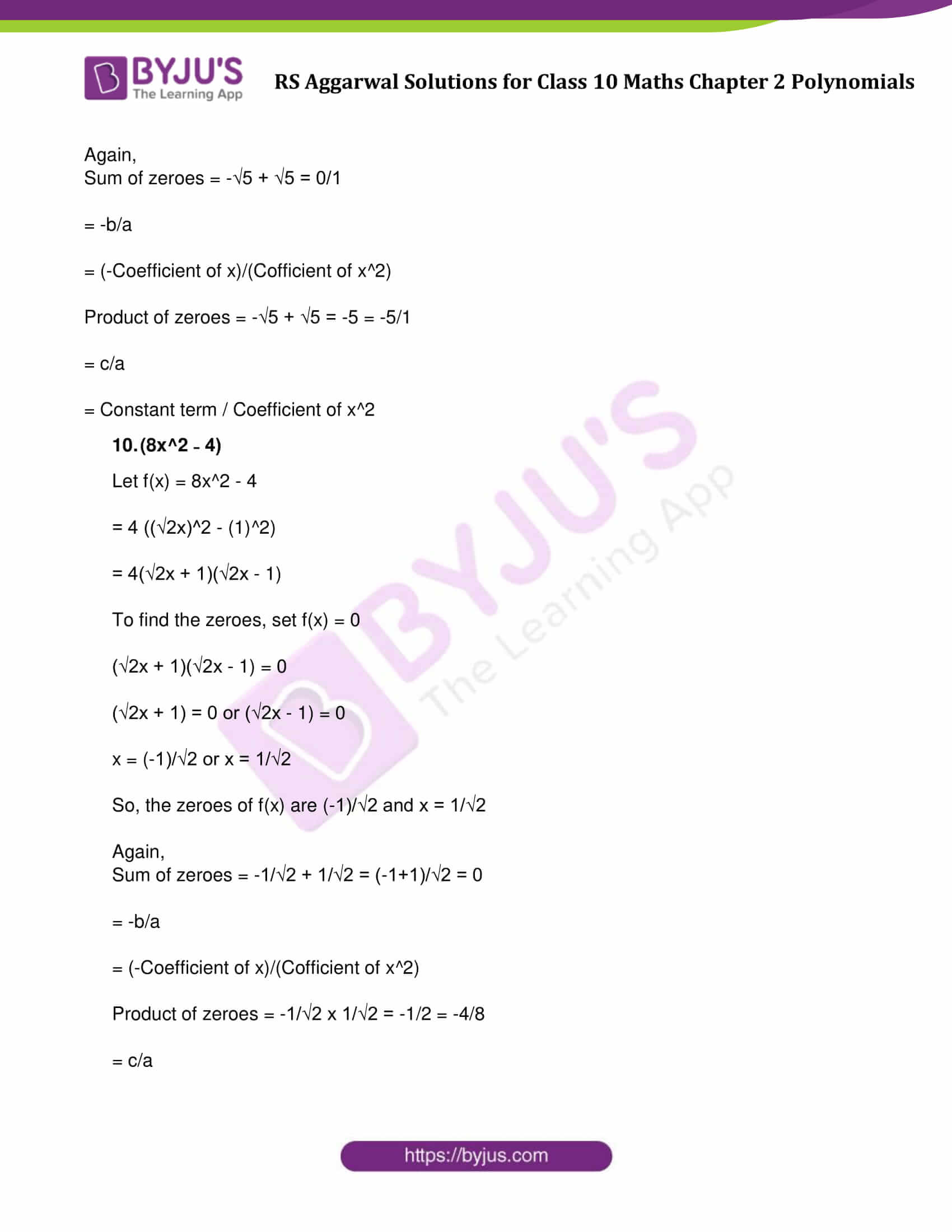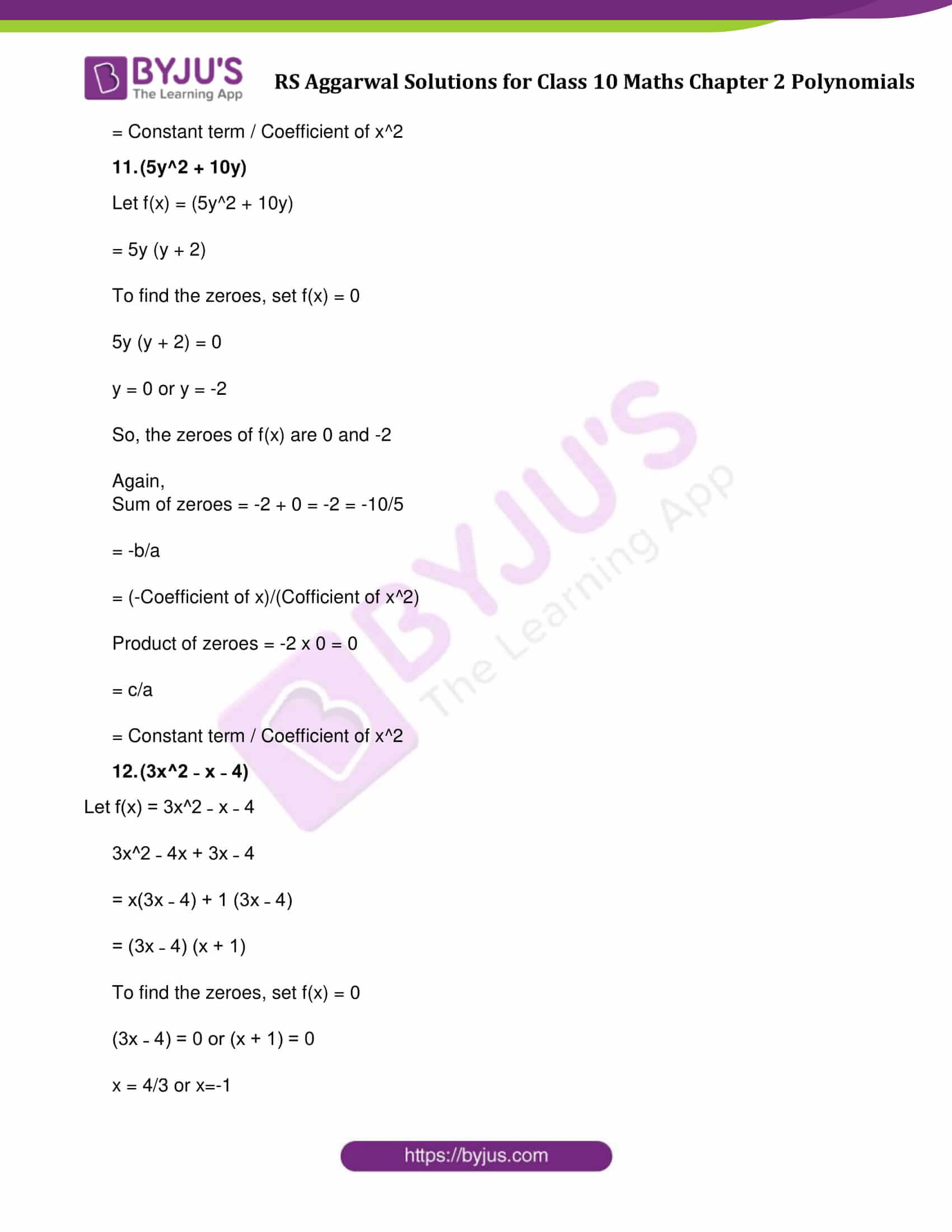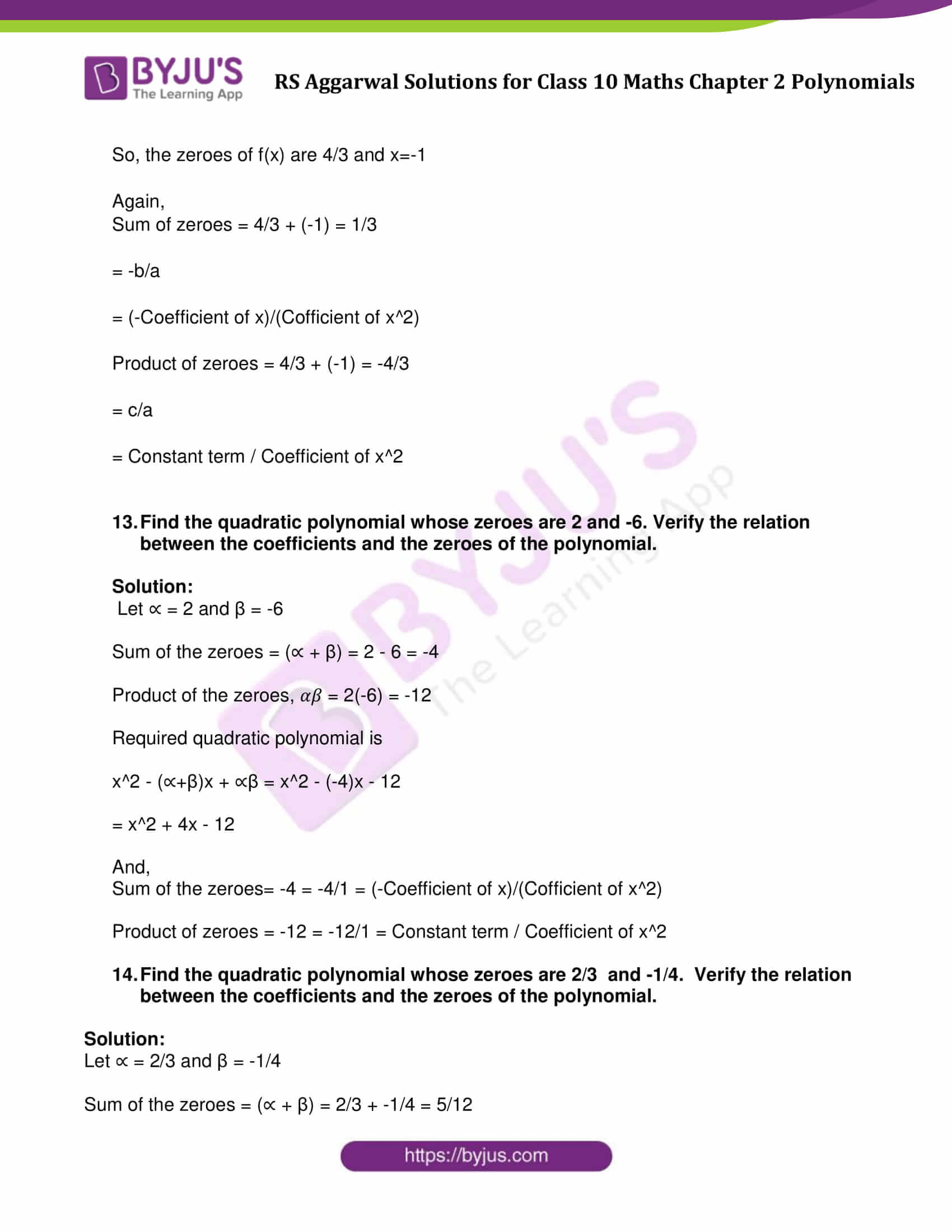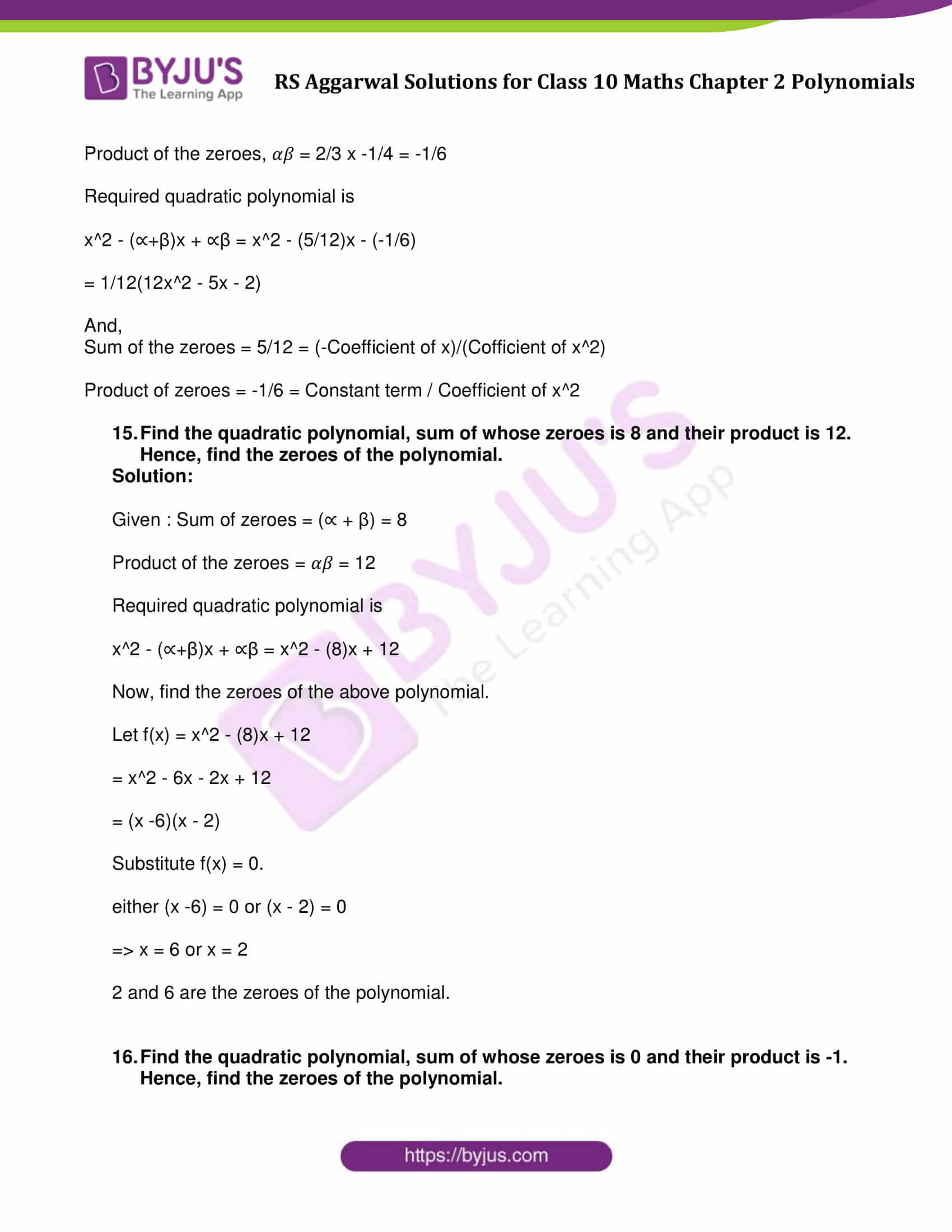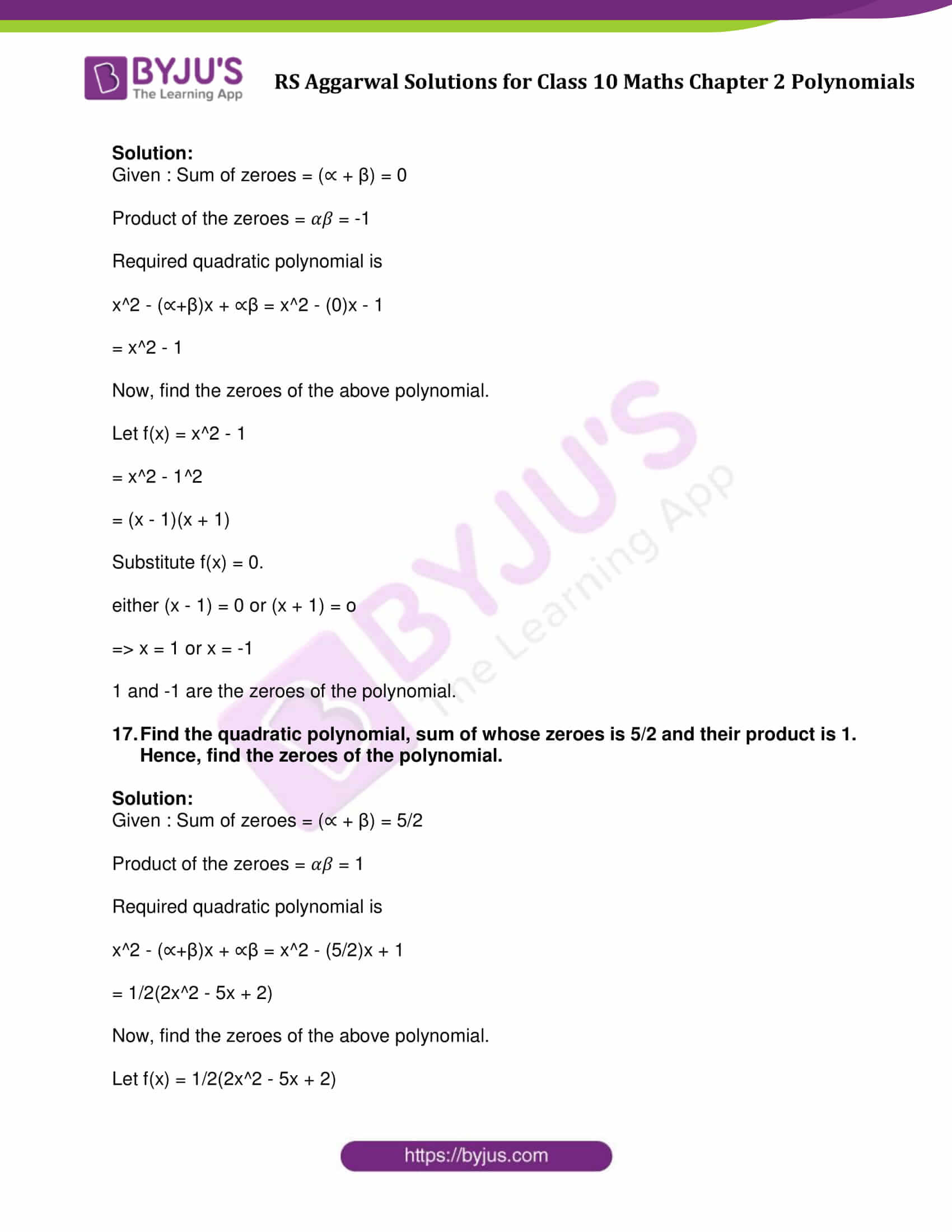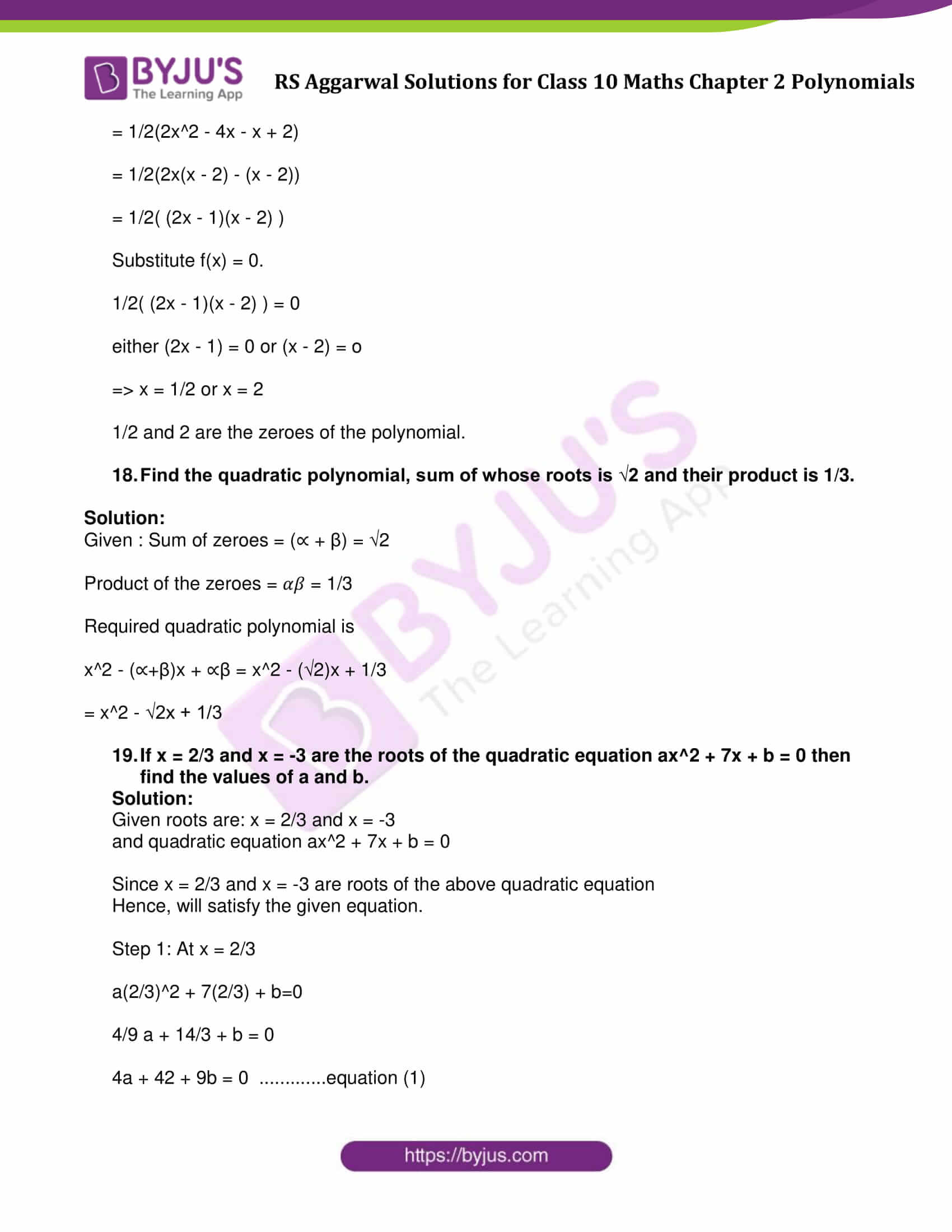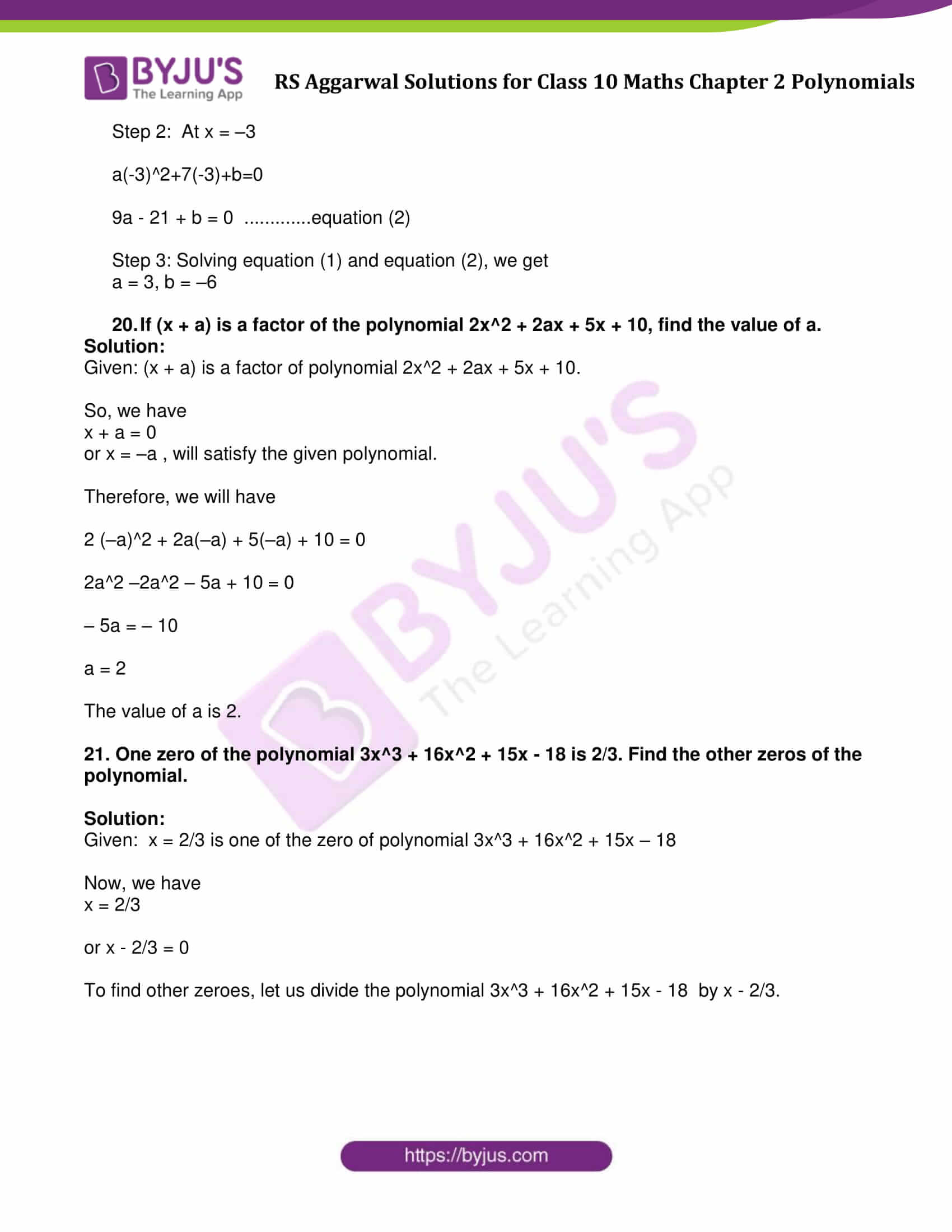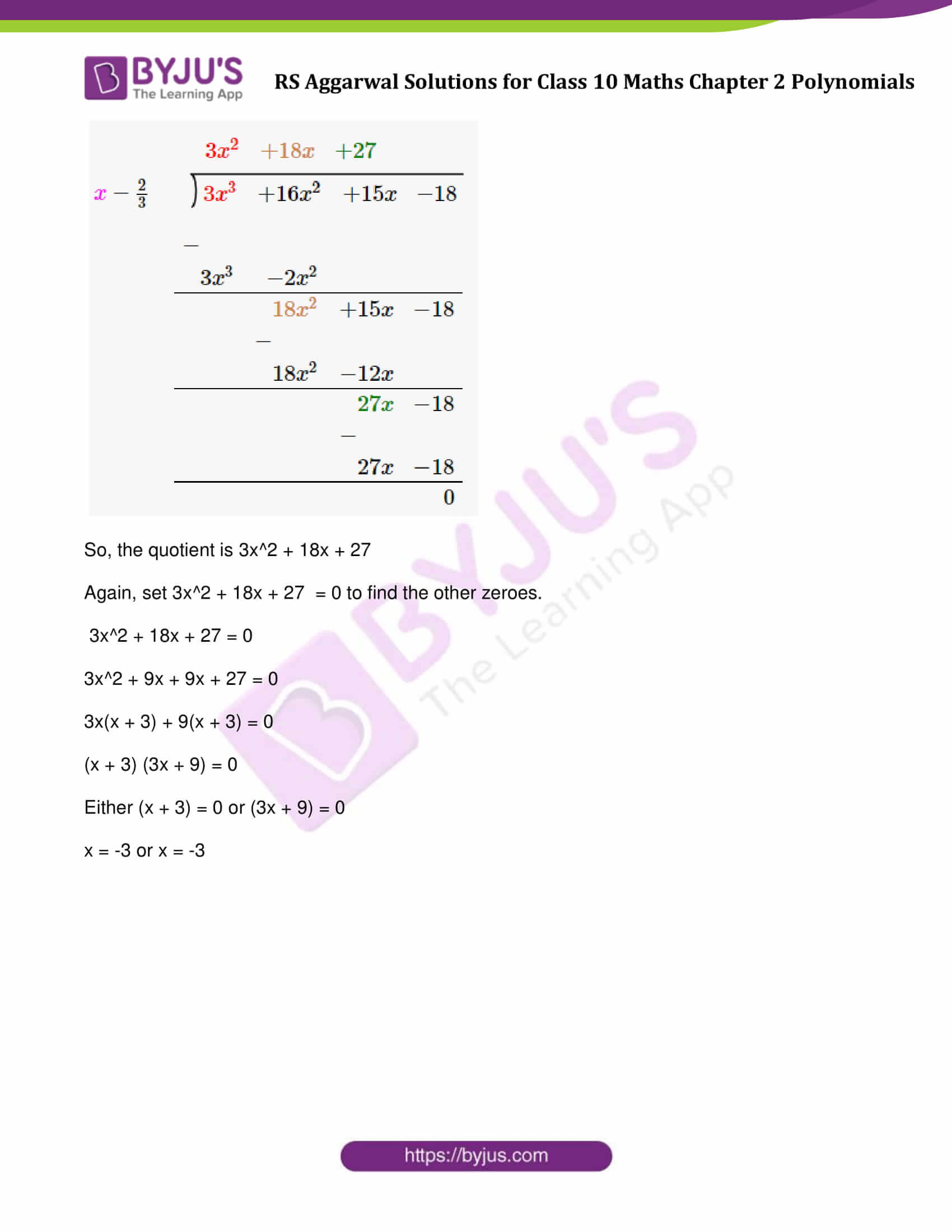### Access other exercise solutions of Class 10 Maths Chapter 2 Polynomials

Exercise 2B Solutions : 19 Questions (Short Answers)

Exercise 2C Solutions : 25 Questions (Short Answers)

## Exercise 2A Page No: 48

Find the zeros of the following quadratic polynomials and verify the relationship between the zeros and the coefficients:

1. x^2 + 7x + 12
2. x^2 ˗ 2x ˗ 8
3. x^2 + 3x ˗ 10
4. 4x^2 ˗ 4x ˗ 3
5. 5x^2 ˗ 4 ˗ 8x
6. 2 √3 x^2 – 5x + √3
7. 2x^2 ˗ 11x + 15
8. 4x^2 – 4x + 1
9. (x^2 ˗ 5)
10. (8x^2 ˗ 4)
11. (5y^2 + 10y)
12. (3x^2 – x – 4)

Solution:

1. x^2 + 7x + 12

Let f(x) = x^2 + 7x + 12

f(x) = x^2 + 4x + 3x + 12

f(x) = x(x+4) + 3(x+4)

f(x) = (x+4)(x+3)

To find the zeroes, set f(x) = 0, then

either (x + 4) = 0 or (x + 3) = 0

x = −4 or x = −3

Again,

Sum of zeroes = (-4 – 3) = -7 = -7/1

= -b/a

= (-Coefficient of x)/(Cofficient of x^2)

Product of zeroes = 12 = 12/1

= c/a

= Constant term / Coefficient of x^2

2. x^2 – 2x – 8

Let f(x) = x^2 ˗ 2x ˗ 8

= x^2 ˗ 4x + 2x ˗ 8

= x(x ˗ 4) + 2(x ˗ 4)

= (x ˗ 4) (x + 2)

To find the zeroes, set f(x) = 0, then

either (x ˗ 4) = 0 or (x+2) = 0

x = 4 or x = -2

Again,

Sum of zeroes = (4 – 2) = 2 = 2/1

= -b/a

= (-Coefficient of x)/(Cofficient of x^2)

Product of zeroes = (4) (-2) = -8/1

= c/a

= Constant term / Coefficient of x^2

3. x^2 + 3x ˗ 10

Let f(x) = x^2 + 3x ˗ 10

= x^2 + 5x ˗ 2x ˗ 10

= x(x + 5) ˗ 2(x + 5)

= (x ˗ 2) (x + 5)

To find the zeroes, set f(x) = 0, then

either x ˗ 2 = 0 or x + 5 = 0

⇒ x = 2 or x = −5.

So, the zeroes of f(x) are 2 and −5.

Again,

Sum of zeroes = 2 + (-5) = -3 = (-3)/1

= -b/a

= (-Coefficient of x)/(Cofficient of x^2)

Product of zeroes = (2)(-5) = -10 = (-10)/1

= c/a

= Constant term / Coefficient of x^2

4. 4x^2 ˗ 4x – 3

Let f(x) = 4x^2 ˗ 4x ˗ 3

= 4x^2 ˗ (6x ˗ 2x) ˗ 3

= 4x^2 ˗ 6x + 2x ˗ 3

= 2x (2x ˗ 3) + 1(2x ˗ 3)

= (2x + 1) (2x ˗ 3)

To find the zeroes, set f(x) = 0

(2x + 1) (2x ˗ 3)= 0

2x + 1 = 0 or 2x ˗ 3 = 0

x = -1/2 or x = 3/2

Again,

Sum of zeroes = (-1/2) + (3/2) = (-1+3)/2 = 2/2

= -b/a

= (-Coefficient of x)/(Cofficient of x^2)

Product of zeroes = (-1/2)(3/2) = (-3)/4

= c/a

= Constant term / Coefficient of x^2

5. 5x^2 ˗ 4 ˗ 8x

Let f(x) = 5x^2 ˗ 4 ˗ 8x

= 5x^2 ˗ 8x ˗ 4

= 5x^2 ˗ (10x ˗ 2x) ˗ 4

= 5x^2 ˗ 10x + 2x ˗ 4

= 5x (x ˗ 2) + 2(x ˗ 2)

= (5x + 2) (x ˗ 2)

To find the zeroes, set f(x) = 0

(5x + 2) (x ˗ 2) = 0

5x + 2 = 0 or x ˗ 2 = 0

x = (−2)/5 or x = 2

Again,

Sum of zeroes = (-2)/5 + 2 = (-2+10)/5 = 8/5

= -b/a

= (-Coefficient of x)/(Cofficient of x^2)

Product of zeroes = (-2/5) x 2 = (-4)/5

= c/a

= Constant term / Coefficient of x^2

6. 2 √3 x^2 – 5x + √3

Let f(x) = 2 √3 x^2 – 5x + √3

= 2 √3 x^2 – 2x – 3x + √3

= 2x(√3x-1) – √3(√3x-1)

To find the zeroes, set f(x) = 0

(√3x – 1) or (2x – √3) = 0

x = 1/√3 = √3/3 or x = √3/2

x = √3/3 or x = √3/2

Again,

Sum of zeroes = 1/√3 + √3/2 = 5/2√3

= (-Coefficient of x)/(Coefficient of x^2)

Product of zeroes = 1/√3 x √3/2 = √3/2√3

= Constant term / Coefficient of x^2

7. 2x^2 – 11x + 15

Let f(x) = 2x^2 ˗ 11x + 15

= 2x^2 ˗ (6x + 5x) + 15

= 2x^2 ˗ 6x ˗ 5x + 15

= 2x (x ˗ 3) ˗ 5 (x ˗ 3)

= (2x ˗ 5) (x ˗ 3)

To find the zeroes, set f(x) = 0

(2x ˗ 5) (x ˗ 3) = 0

2x ˗ 5= 0 or x ˗ 3 = 0

x = 5/2 or x = 3

Again,

Sum of zeroes = 5/2 + 3 = (5+6)/2 = 11/2

= -b/a

= (-Coefficient of x)/(Cofficient of x^2)

Product of zeroes = 5/2 x 3 = 15/2

= c/a

= Constant term / Coefficient of x^2

8. 4x^2 ˗ 4x + 1

Let f(x) = 4x^2 ˗ 4x + 1

= (2x^2) – 2(2x)(1) + (1)^2

= (2x – 1)^2

To find the zeroes, set f(x) = 0

(2x – 1)^2 = 0

x = 1/2 or x = 1/2

Again,

Sum of zeroes = 1/2+1/2=1=1/1

= -b/a

= (-Coefficient of x) / (Cofficient of x^2)

Product of zeroes = 1/2 x 1/2=1/4

= c/a

= Constant term / Coefficient of x^2

9. (x^2 – 5)

Let f(x) = (x^2 – 5)

= (x^2-(√5)^2)

= (x + √5)(x – √5)

To find the zeroes, set f(x) = 0

(x + √5)(x – √5)=0

x = -√5 or x = √5

Again,

Sum of zeroes = -√5 + √5 = 0/1

= -b/a

= (-Coefficient of x)/(Cofficient of x^2)

Product of zeroes = -√5 + √5 = -5 = -5/1

= c/a

= Constant term / Coefficient of x^2

10. (8x^2 ˗ 4)

Let f(x) = 8x^2 – 4

= 4 ((√2x)^2 – (1)^2)

= 4(√2x + 1)(√2x – 1)

To find the zeroes, set f(x) = 0

(√2x + 1)(√2x – 1) = 0

(√2x + 1) = 0 or (√2x – 1) = 0

x = (-1)/√2 or x = 1/√2

So, the zeroes of f(x) are (-1)/√2 and x = 1/√2

Again,

Sum of zeroes = -1/√2 + 1/√2 = (-1+1)/√2 = 0

= -b/a

= (-Coefficient of x)/(Cofficient of x^2)

Product of zeroes = -1/√2 x 1/√2 = -1/2 = -4/8

= c/a

= Constant term / Coefficient of x^2

11. (5y^2 + 10y)

Let f(x) = (5y^2 + 10y)

= 5y (y + 2)

To find the zeroes, set f(x) = 0

5y (y + 2) = 0

y = 0 or y = -2

So, the zeroes of f(x) are 0 and -2

Again,

Sum of zeroes = -2 + 0 = -2 = -10/5

= -b/a

= (-Coefficient of x)/(Cofficient of x^2)

Product of zeroes = -2 x 0 = 0

= c/a

= Constant term / Coefficient of x^2

12. (3x^2 ˗ x ˗ 4)

Let f(x) = 3x^2 ˗ x ˗ 4

3x^2 ˗ 4x + 3x ˗ 4

= x(3x ˗ 4) + 1 (3x ˗ 4)

= (3x ˗ 4) (x + 1)

To find the zeroes, set f(x) = 0

(3x ˗ 4) = 0 or (x + 1) = 0

⇨ x = 4/3 or x=-1

So, the zeroes of f(x) are 4/3 and x=-1

Again,

Sum of zeroes = 4/3 + (-1) = 1/3

= -b/a

= (-Coefficient of x)/(Cofficient of x^2)

Product of zeroes = 4/3 + (-1) = -4/3

= c/a

= Constant term / Coefficient of x^2

13. Find the quadratic polynomial whose zeroes are 2 and -6. Verify the relation between the coefficients and the zeroes of the polynomial.

Solution:

Let ∝ = 2 and β = -6

Sum of the zeroes = (∝ + β) = 2 – 6 = -4

Product of the zeroes, 𝛼𝛽 = 2(-6) = -12

⇨ x^2 – (∝+β)x + ∝β = x^2 – (-4)x – 12

= x^2 + 4x – 12

And,

Sum of the zeroes = – 4 = -4/1 = (-Coefficient of x)/(Cofficient of x^2)

Product of zeroes = -12 = -12/1 = Constant term / Coefficient of x^2

14. Find the quadratic polynomial whose zeroes are 2/3 and -1/4. Verify the relation between the coefficients and the zeroes of the polynomial.

Solution:

Let ∝ = 2/3 and β = -1/4

Sum of the zeroes = (∝ + β) = 2/3 + -1/4 = 5/12

Product of the zeroes, 𝛼𝛽 = 2/3 x -1/4 = -1/6

x^2 – (∝+β)x + ∝β = x^2 – (5/12)x – (-1/6)

= 1/12(12x^2 – 5x – 2)

And,

Sum of the zeroes = 5/12 = (-Coefficient of x)/(Coefficient of x^2)

Product of zeroes = -1/6 = Constant term / Coefficient of x^2

15. Find the quadratic polynomial, sum of whose zeroes is 8 and their product is 12. Hence, find the zeroes of the polynomial.

Solution:

Given : Sum of zeroes = (∝ + β) = 8

Product of the zeroes = 𝛼𝛽 = 12

x^2 – (∝+β)x + ∝β = x^2 – (8)x + 12

Now, find the zeroes of the above polynomial.

Let f(x) = x^2 – (8)x + 12

= x^2 – 6x – 2x + 12

= (x -6)(x – 2)

Substitute f(x) = 0.

either (x -6) = 0 or (x – 2) = 0

⇨ x = 6 or x = 2

2 and 6 are the zeroes of the polynomial.

16. Find the quadratic polynomial, sum of whose zeroes is 0 and their product is -1. Hence, find the zeroes of the polynomial.

Solution:

Given : Sum of zeroes = (∝ + β) = 0

Product of the zeroes = 𝛼𝛽 = -1

x^2 – (∝+β)x + ∝β = x^2 – (0)x – 1

= x^2 – 1

Now, find the zeroes of the above polynomial.

Let f(x) = x^2 – 1

= x^2 – 1^2

= (x – 1)(x + 1)

Substitute f(x) = 0.

either (x – 1) = 0 or (x + 1) = o

⇨ x = 1 or x = -1

1 and -1 are the zeroes of the polynomial.

17. Find the quadratic polynomial, sum of whose zeroes is 5/2 and their product is 1. Hence, find the zeroes of the polynomial.

Solution:

Given : Sum of zeroes = (∝ + β) = 5/2

Product of the zeroes = 𝛼𝛽 = 1

x^2 – (∝+β)x + ∝β = x^2 – (5/2)x + 1

= 1/2(2x^2 – 5x + 2)

Now, find the zeroes of the above polynomial.

Let f(x) = 1/2(2x^2 – 5x + 2)

= 1/2(2x^2 – 4x – x + 2)

= 1/2(2x(x – 2) – (x – 2))

= 1/2( (2x – 1)(x – 2) )

Substitute f(x) = 0.

1/2( (2x – 1)(x – 2) ) = 0

either (2x – 1) = 0 or (x – 2) = o

⇨ x = 1/2 or x = 2

1/2 and 2 are the zeroes of the polynomial.

18. Find the quadratic polynomial, sum of whose roots is √2 and their product is 1/3.

Solution:

Given : Sum of zeroes = (∝ + β) = √2

Product of the zeroes = 𝛼𝛽 = 1/3

x^2 – (∝+β)x + ∝β = x^2 – (√2)x + 1/3

= x^2 – √2x + 1/3

19. If x = 2/3 and x = -3 are the roots of the quadratic equation ax^2 + 7x + b = 0 then find the values of a and b.

Solution:

Given roots are: x = 2/3 and x = -3

and quadratic equation ax^2 + 7x + b = 0

Since x = 2/3 and x = -3 are roots of the above quadratic equation

Hence, will satisfy the given equation.

Step 1: At x = 2/3

a(2/3)^2 + 7(2/3) + b=0

4/9 a + 14/3 + b = 0

4a + 42 + 9b = 0 ………….equation (1)

Step 2: At x = –3

a(-3)^2+7(-3)+b=0

9a – 21 + b = 0 ………….equation (2)

Step 3: Solving equation (1) and equation (2), we get

a = 3, b = –6

20. If (x + a) is a factor of the polynomial 2x^2 + 2ax + 5x + 10, find the value of a.

Solution:

Given: (x + a) is a factor of polynomial 2x^2 + 2ax + 5x + 10.

So, we have

x + a = 0

or x = –a , will satisfy the given polynomial.

Therefore, we will have

2 (–a)^2 + 2a(–a) + 5(–a) + 10 = 0

2a^2 –2a^2 – 5a + 10 = 0

– 5a = – 10

a = 2

The value of a is 2.

21. One zero of the polynomial 3x^3 + 16x^2 + 15x – 18 is 2/3. Find the other zeros of the polynomial.

Solution:

Given: x = 2/3 is one of the zero of polynomial 3x^3 + 16x^2 + 15x – 18

Now, we have

x = 2/3

or x – 2/3 = 0

To find other zeroes, let us divide the polynomial 3x^3 + 16x^2 + 15x – 18 by x – 2/3.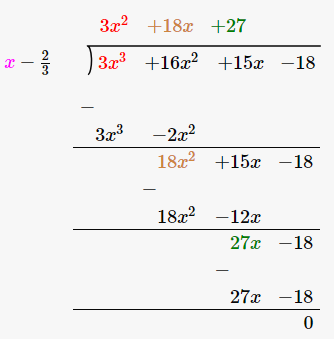So, the quotient is 3x^2 + 18x + 27

Again, set 3x^2 + 18x + 27 = 0 to find the other zeroes.

3x^2 + 18x + 27 = 0

3x^2 + 9x + 9x + 27 = 0

3x(x + 3) + 9(x + 3) = 0

(x + 3) (3x + 9) = 0

Either (x + 3) = 0 or (3x + 9) = 0

x = -3 or x = -3

## RS Aggarwal Solutions for Class 10 Maths Chapter 2 Polynomials Exercise 2A

Class 10 Maths Chapter 2 Polynomials Exercise 2A is based on the following topics and subtopics:

• Linear Polynomials